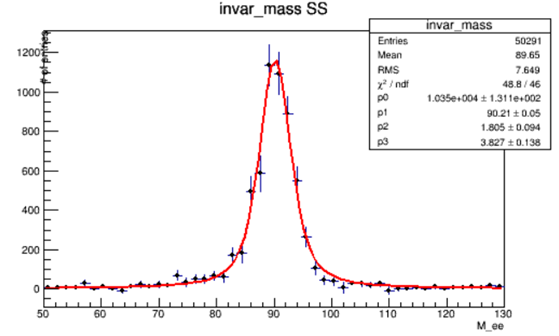# Histograms fitting using likelihood fit

Dear Rootians,

I am trying to fit the histogram for invariant mass of two leptons (Z->ee) with likelihood fit.
I have two different distribution based on sign of electron i.e Same-Sign(SS) and Opposite Sign(OS).
I have tried different function e.g Gaussian+polynomial, Lorentzian, Voigt, DS-CB etc.
For Same Sign distribution, got good fit with Voigt function.The function and results:

``````root  TF1 *f1 = new TF1("f1","( * TMath::Voigt(x - , , , 4))", 50,130);
root  f1->SetParameters(10000, 90,2,3);
root  invar_mass->Fit("f1","WLR", "ep");

****************************************
Minimizer is Minuit / Migrad
MinFCN                    =      272.163
Chi2                      =      48.8082
NDf                       =           46
Edm                       = 7.15068e-008
NCalls                    =           91
p0                        =        10345   +/-   425.092
p1                        =       90.205   +/-   0.162891
p2                        =      1.80446   +/-   0.374657
p3                        =      3.82712   +/-   0.528939

``````

But for OS distribution the Chi2 value is very high. With same function

``````root  TF1 *f1 = new TF1("f1","( * TMath::Voigt(x - , , , 4))", 50,130);
root  f1->SetParameters(26000000, 90,0.001,4);
root  invar_mass->Fit("f1","WLR", "ep");
Warning in <ROOT::Math::Fitter::ApplyWeightCorrection>: Covariance matrix for weighted likelihood is not accurate, the errors may be not reliable
Error in <TMinuitMinimizer::GetHessianMatrix>: Hessian matrix has not been computed - status 1
Error in <ROOT::Math::Fitter::ApplyWeightCorrection>: Error retrieving Hesse on weight2 likelihood - cannot compute errors
Warning in <Fit>: Abnormal termination of minimization.

****************************************
Minimizer is Minuit / Migrad
MinFCN                    =       162202
Chi2                      =      22154.4
NDf                       =           46
Edm                       = 1.42054e-011
NCalls                    =          102
p0                        = 2.61392e+007   +/-   6592.51
p1                        =      90.8288   +/-   0.000865041
p2                        =   0.00102603   +/-   1.22141e-008
p3                        =      4.68913   +/-   0.00162434
``````

Also tried with Lorentzian function …Which give same chi2 value

``````root  TF1 *f1 = new TF1("f1","((0.5**/TMath::Pi()) / TMath::Max( 1.e-10,(x-)*(x-) + .25**))", 10,170);
root  f1->SetParameters(2.61516e+07, 4,90);
root  invar_mass->Fit("f1","WLR", "ep");
Warning in <ROOT::Math::Fitter::ApplyWeightCorrection>: Covariance matrix for weighted likelihood is not accurate, the errors may be not reliable
Warning in <ROOT::Math::Fitter::ApplyWeightCorrection>: Covariance matrix for weighted likelihood was forced to be defined positive

****************************************
Minimizer is Minuit / Migrad
MinFCN                    =       162202
Chi2                      =      22154.4
NDf                       =           47
Edm                       = 1.75801e-010
NCalls                    =           78
p0                        = 2.61392e+007   +/-   61291.9
p1                        =      4.68913   +/-   0.0141233
p2                        =      90.8288   +/-   0.0078541
``````

Then I tried double-sided CB function to fit the OS distribution. The fit results are without any error or warning, but still have the Chi2 = 1044.79

``````****************************************
Minimizer is Minuit / Migrad
MinFCN                    =      8047.47
Chi2                      =      1044.79
NDf                       =           42
Edm                       =  5.06269e-09
NCalls                    =          801
p0                        =  3.11001e+06   +/-   7191.01
p1                        =      90.8832   +/-   0.00604309
p2                        =      2.64637   +/-   0.0170922
p3                        =      1.31658   +/-   0.019208
p4                        =       2.4644   +/-   0.116622
p5                        =      1.45723   +/-   0.0616649
p6                        =      2.63389   +/-   0.307276
p7                        =      8530.86   +/-   1179
``````

Dose the last fit (with DS-CB) gives the good results? Or should I try to fit the Opposite- Sign distribution with some other function? although the fit result are shown without any error/ warning but Chi2 value is still very high. Or I need to check for some other test than the Chi2 to check the fit is good or not?

Many thanks
(I have also attached the histogram for SS and OS)
Zjets_SS.root (4.4 KB)
zjets_OS.root (4.4 KB)

@moneta Can you comment?

In principle, you will get some improvement if you use the “I” fit option.

However, I think you need a better “model” for your “OS” data (i.e., a function which can “describe” it). You need to discuss it with your colleagues and / or supervisor.

1 Like

Hi @Wile_E_Coyote ,
Thanks for your considerationYou are right… by including the “I” fit option

``````TFitResultPtr r = h_mee->Fit(dcb,"SIRWLV+", "ep");
``````

the Chi2 improve form 1044.79 → 919.53
I have tried many model function, e.g Gaussian+polynomial, Lorentzian+ plol0, Voigt, DS-CB.
There are lot more statistic in “OS” distribution i.e order of 1000 than SS, Dose this also effect the Chi2?
Thanks

I think, roughly speaking, 1000 times more statistics means that you are sqrt(1000) times more sensitive to the shape of the distribution.

BTW. In order to get a better plot of the “f1” fit function, add: `f1->SetNpx(250);`

This topic was automatically closed 14 days after the last reply. New replies are no longer allowed.